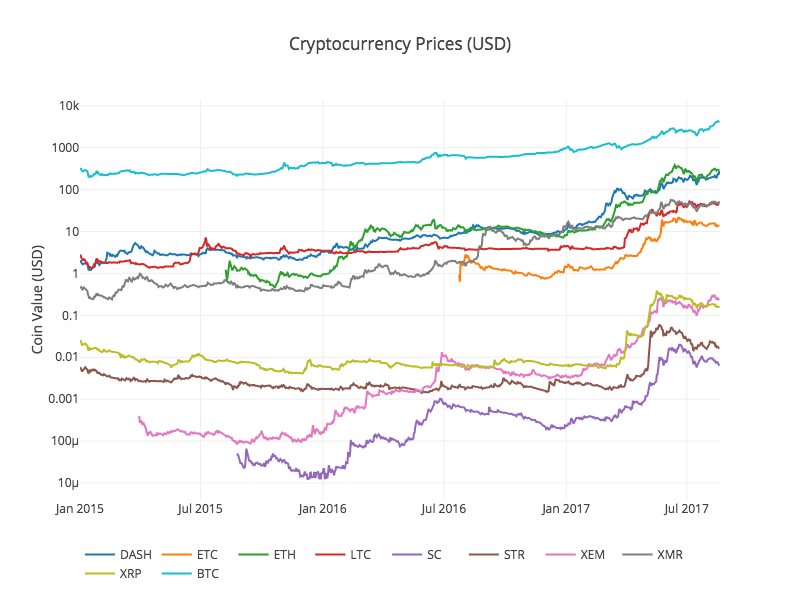# A Concrete Introduction to Probability (using Python)

This notebook covers the basics of probability theory, with Python 3 implementations. (You should have some background in probability and Python.)

In 1814, Pierre-Simon Laplace wrote:

Probability … is thus simply a fraction whose numerator is the number of favorable cases and whose denominator is the number of all the cases possible … when nothing leads us to expect that any one of these cases should occur more than any other.Laplace really nailed it, way back then! If you want to untangle a probability problem, all you have to do is be methodical about defining exactly what the cases are, and then careful in counting the number of favorable and total cases. We’ll start being methodical by defining some vocabulary:

• Experiment: An occurrence with an uncertain outcome that we can observe.
For example, rolling a die.
• Outcome: The result of an experiment; one particular state of the world. What Laplace calls a “case.”
For example: `4`.
• Sample Space: The set of all possible outcomes for the experiment.
For example, `{1, 2, 3, 4, 5, 6}`.
• Event: A subset of possible outcomes that together have some property we are interested in.
For example, the event “even die roll” is the set of outcomes `{2, 4, 6}`.
• Probability: As Laplace said, the probability of an event with respect to a sample space is the number of favorable cases (outcomes from the sample space that are in the event) divided by the total number of cases in the sample space. (This assumes that all outcomes in the sample space are equally likely.) Since it is a ratio, probability will always be a number between 0 (representing an impossible event) and 1 (representing a certain event).
For example, the probability of an even die roll is 3/6 = 1/2.

This notebook will develop all these concepts; I also have a second part that covers paradoxes in Probability Theory.

http://nbviewer.jupyter.org/url/norvig.com/ipython/Probability.ipynb

# Understanding word vectors

… for, like, actual poets. By Allison Parrish

In this tutorial, I’m going to show you how word vectors work. This tutorial assumes a good amount of Python knowledge, but even if you’re not a Python expert, you should be able to follow along and make small changes to the examples without too much trouble.

This is a “Jupyter Notebook,” which consists of text and “cells” of code. After you’ve loaded the notebook, you can execute the code in a cell by highlighting it and hitting Ctrl+Enter. In general, you need to execute the cells from top to bottom, but you can usually run a cell more than once without messing anything up. Experiment!

https://gist.github.com/aparrish/2f562e3737544cf29aaf1af30362f469

# ANALYZING CRYPTOCURRENCY MARKETS USING PYTHON

## A DATA-DRIVEN APPROACH TO CRYPTOCURRENCY SPECULATION

How do Bitcoin markets behave? What are the causes of the sudden spikes and dips in cryptocurrency values? Are the markets for different altcoins inseparably linked or largely independent? How can we predict what will happen next?

Articles on cryptocurrencies, such as Bitcoin and Ethereum, are rife with speculation these days, with hundreds of self-proclaimed experts advocating for the trends that they expect to emerge. What is lacking from many of these analyses is a strong foundation of data and statistics to backup the claims.

The goal of this article is to provide an easy introduction to cryptocurrency analysis using Python. We will walk through a simple Python script to retrieve, analyze, and visualize data on different cryptocurrencies. In the process, we will uncover an interesting trend in how these volatile markets behave, and how they are evolving.This is not a post explaining what cryptocurrencies are (if you want one, I would recommend this great overview), nor is it an opinion piece on which specific currencies will rise and which will fall. Instead, all that we are concerned about in this tutorial is procuring the raw data and uncovering the stories hidden in the numbers.

# Pandas and Python Real World Project (GPS data)

“To celebrate my sub-30 minute finish on the manitou springs incline, I figured I’d drop some python and pandas knowledge while analyzing the data using beautifulsoup, pandas, python dateutil, python googlemaps, python geopy and the standard library.

I try to look at things in a non-trivial way and reflective of actual problems you encounter analyzing real world data. The data file is here https://gist.github.com/jrjames83/4de9d124e5f43a61be9cb2a… come code along!”